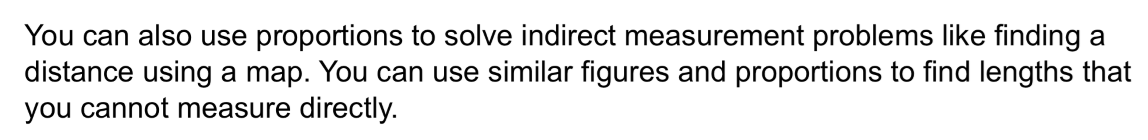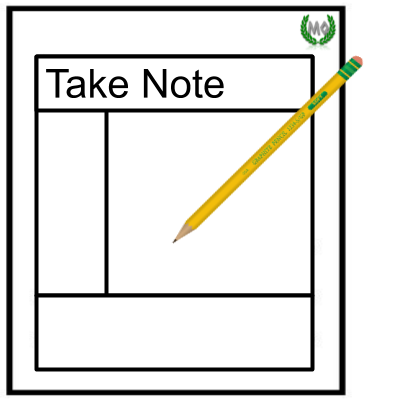Algebra 1 2-8 Guided Practice: Proportions and Similar Figures
starstarstarstarstarstarstarstarstarstar
by Matthew Richardson
| 18 Questions1
2
1
10
Solve It! A sailboat and an accurate model of the boat are shown. How many feet long is the model sailboat? Enter only a number.
2
103
4
6
7
5
8
3
10
Take Note: Define similar figures.
4
10
Take Note: Create an example pair of similar figures on the canvas.
5
5
Take Note: Type the symbol for similar to.
6
10
Take Note: What does it mean for angles to be congruent?
7
10
Take Note: Create an example pair of congruent angles on the canvas.
8
5
Take Note: Type the symbol for congruent to.9
9
10
Problem 1 Got It?
A
B
C
D10
30
Problem 2 Got It? Create a diagram on the canvas to represent the scenario. Remember that you can insert pictures of objects -- like a man, a flagpole, a shadow, etc. -- instead of drawing them all. Be sure to label all important information.11
11
10
Problem 2 Got It? A man who is 6 ft tall is standing next to a flagpole. The shadow of the man is 3.5 ft and the shadow of the flagpole is 17.5 ft. What is the height of the flagpole?
A
B
C
D12
13
12
10
Take Note: Define scale drawing.
13
10
Take Note: Define scale [of a scale drawing].14
14
10
Problem 3 Got It? The distance from Jacksonville to Gainsville on the map is about 0.6 in. What is the actual distance from Jacksonville to Gainsville?16
16
10
Take Note: Define scale model.
17
10
Problem 4 Got It? A scale model of a building is 6 in. tall. The scale of the model is 1 in. : 50 ft. How tall is the building?
300 ft
3600 ft
8.33 ft
100 ft18
18
10
Take Note: Summarize the mathematical content of this lesson. What topics, ideas, and vocabulary were introduced?